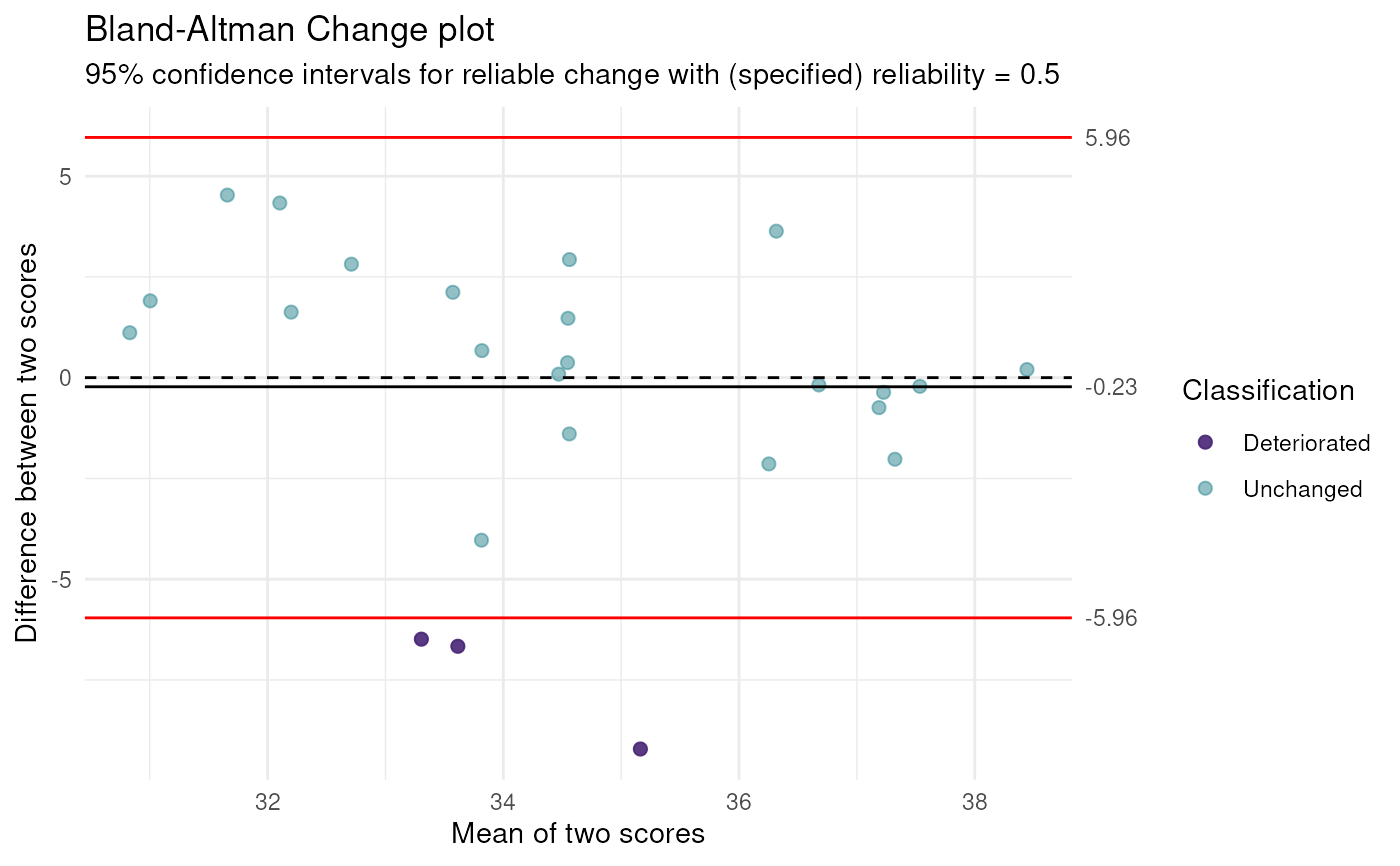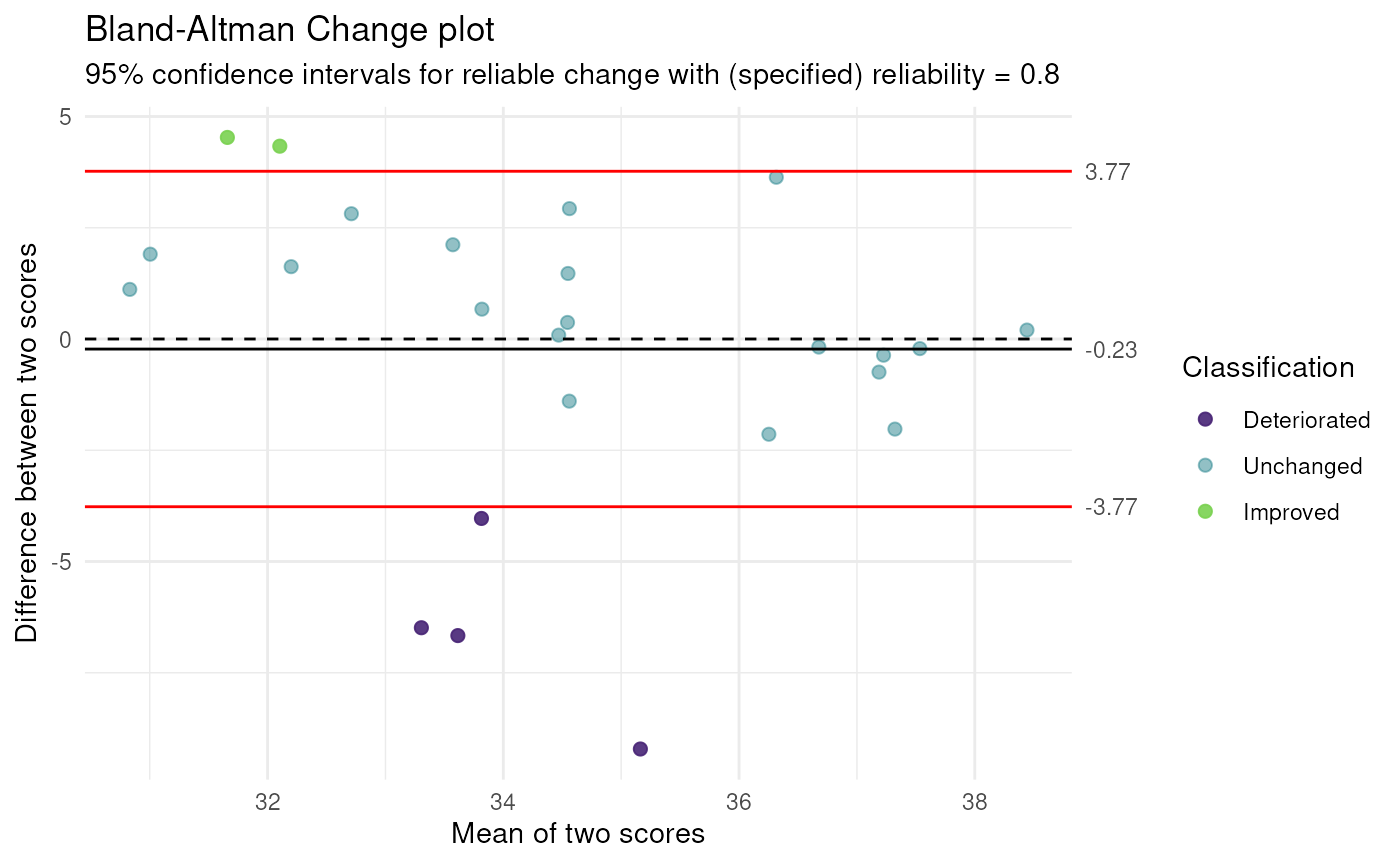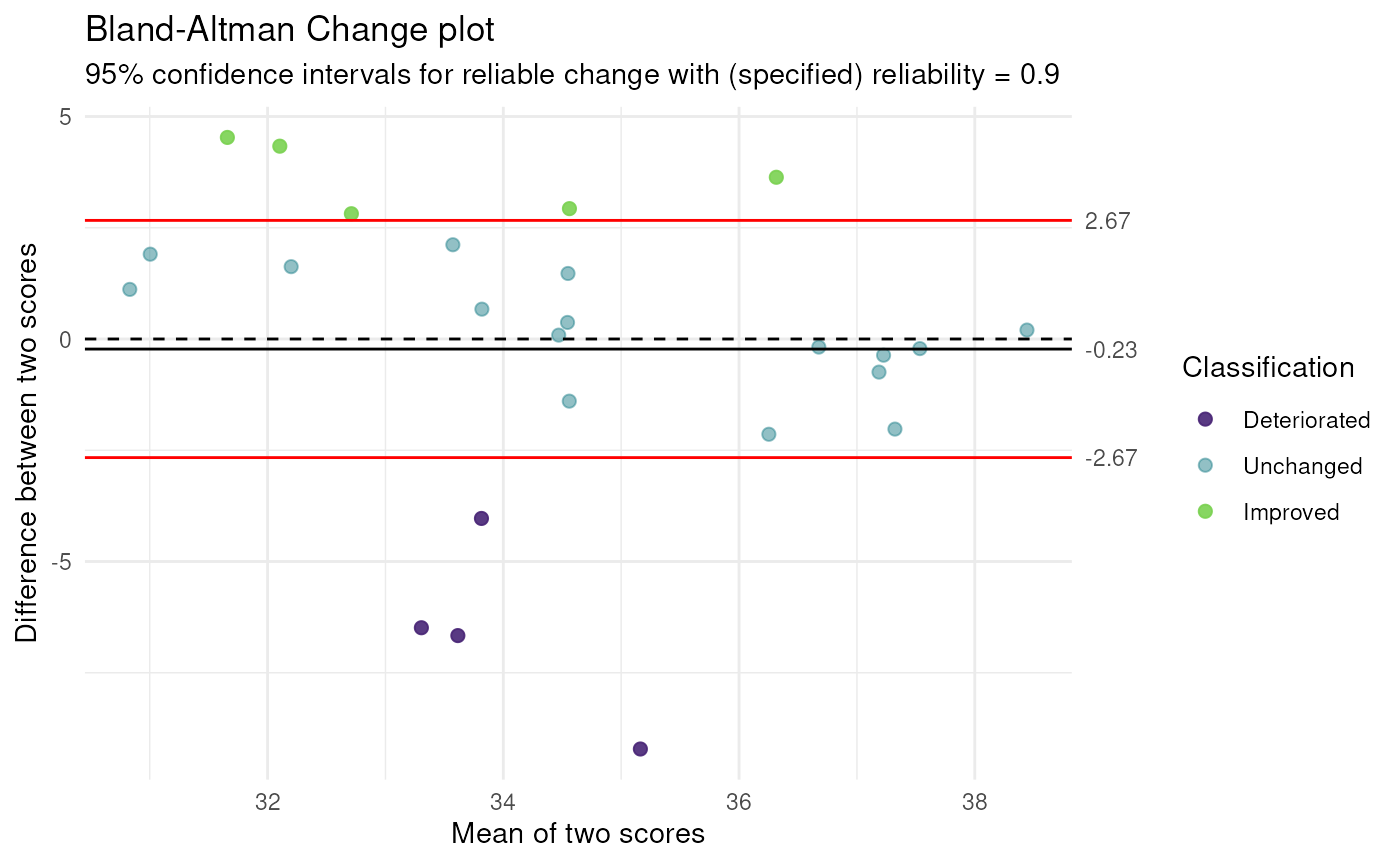Bland-Altman Change plot

BAC_plot(
data,
cols = names(data),
reliability = NULL,
pointSize = 2,
deterioratedColor = "#482576E6",
unchangedColor = "#25848E80",
zeroLineColor = "black",
zeroLineType = "dashed",
ciLineColor = "red",
ciLineType = "solid",
conf.level = 0.95,
theme = ggplot2::theme_minimal(),
ignoreBias = FALSE,
iccFromPsych = FALSE,
iccFromPsychArgs = NULL
)

## Arguments

data

The data frame; if it only has two columns, the first of which is the pre-change column, cols can be left empty.

cols

The names of the columns with the data; the first is the column with the pre-change data, the second the column after the change.

reliability

The reliability estimate, for example as obtained with the ICC() function in the psych() package; can be omitted, in which case the intraclass correlation is computed.

pointSize

The size of the points in the plot.

deterioratedColor, unchangedColor, improvedColor

The colors to use for cases who deteriorate, stay the same, and improve, respectively.

zeroLineColor, ciLineColor

The colors for the line at 0 (no change) and at the confidence interval bounds (i.e. the point at which a difference becomes indicative of change given the reliability), respectively.

zeroLineType, ciLineType

The line types for the line at 0 (no change) and at the confidence interval bounds (i.e. the point at which a difference becomes indicative of change given the reliability), respectively.

conf.level

The confidence level of the confidence interval.

theme

The ggplot2 theme to use.

ignoreBias

Whether to ignore bias (i.e. allow the measurements at the second time to shift upwards or downwards). If FALSE, the variance associated with such a shift is considered error variance (i.e. 'unreliability').

iccFromPsych

Whether to compute ICC using the psych::ICC() function or not.

iccFromPsychArgs

If using the psych::ICC() function, the arguments to pass.

A ggplot2 plot.

## Examples

### Create smaller dataset for example
dat <-
ufs::testRetestSimData[
1:25,
c('t0_item1', 't1_item1')
];

ufs::BAC_plot(dat, reliability = .5);ufs::BAC_plot(dat, reliability = .8);ufs::BAC_plot(dat, reliability = .9);